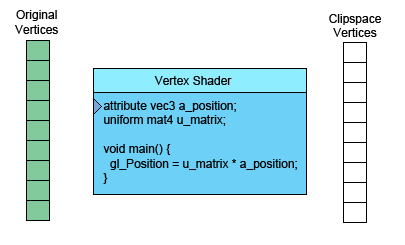# WebGLFundamentals.org

Fix, Fork, Contribute

# WebGL 工作原理

``````var primitiveType = gl.TRIANGLES;
var offset = 0;
var count = 9;
gl.drawArrays(primitiveType, offset, count);
````````````// 定义一个三角形填充到缓冲里
function setGeometry(gl) {
gl.bufferData(
gl.ARRAY_BUFFER,
new Float32Array([
0, -100,
150,  125,
-175,  100]),
gl.STATIC_DRAW);
}
``````

``````// 绘制场景
function drawScene() {
...
// 绘制几何体
var primitiveType = gl.TRIANGLES;
var offset = 0;
var count = 3;
gl.drawArrays(primitiveType, offset, count);
}
``````

``````varying vec4 v_color;
...
void main() {
// 将位置和矩阵相乘
gl_Position = vec4((u_matrix * vec3(a_position, 1)).xy, 0, 1);

// 从裁减空间转换到颜色空间
// 裁减空间范围 -1.0 到 +1.0
// 颜色空间范围 0.0 到 1.0
*  v_color = gl_Position * 0.5 + 0.5;
}
``````

``````precision mediump float;

*varying vec4 v_color;

void main() {
*  gl_FragColor = v_color;
}
``````

WebGL会将同名的可变量从顶点着色器输入到片断着色器中。

WebGL先获得顶点着色器中计算的三个颜色值，在光栅化三角形时将会根据这三个值进行插值。 每一个像素在调用片断着色器时，可变量的值是与之对应的插值。

0-100
150125
-175100

0.0000.660
0.750-0.830
-0.875-0.660

0.50000.8300.5
0.87500.0860.5
0.06250.1700.5

v_color is interpolated between v0, v1 and v2

``````attribute vec2 a_position;
+attribute vec4 a_color;
...
varying vec4 v_color;

void main() {
...
// 直接把属性值中的数据赋给可变量
*  v_color = a_color;
}
``````

``````  // 寻找顶点着色器中需要的数据
var positionLocation = gl.getAttribLocation(program, "a_position");
+  var colorLocation = gl.getAttribLocation(program, "a_color");
...
+  // 给颜色数据创建一个缓冲
+  var colorBuffer = gl.createBuffer();
+  gl.bindBuffer(gl.ARRAY_BUFFER, colorBuffer);
+  // 设置颜色
+  setColors(gl);
...

+// 给矩形的两个三角形
+// 设置颜色值并发到缓冲
+function setColors(gl) {
+  // 生成两个随机颜色
+  var r1 = Math.random();
+  var b1 = Math.random();
+  var g1 = Math.random();
+
+  var r2 = Math.random();
+  var b2 = Math.random();
+  var g2 = Math.random();
+
+  gl.bufferData(
+      gl.ARRAY_BUFFER,
+      new Float32Array(
+        [ r1, b1, g1, 1,
+          r1, b1, g1, 1,
+          r1, b1, g1, 1,
+          r2, b2, g2, 1,
+          r2, b2, g2, 1,
+          r2, b2, g2, 1]),
+      gl.STATIC_DRAW);
+}
``````

``````+gl.enableVertexAttribArray(colorLocation);
+
+// 绑定颜色缓冲
+gl.bindBuffer(gl.ARRAY_BUFFER, colorBuffer);
+
+// 告诉颜色属性怎么从 colorBuffer (ARRAY_BUFFER) 中读取颜色值
+var size = 4;          // 每次迭代使用4个单位的数据
+var type = gl.FLOAT;   // 单位数据类型是32位的浮点型
+var normalize = false; // 不需要归一化数据
+var stride = 0;        // 0 = 移动距离 * 单位距离长度sizeof(type)
// 每次迭代跳多少距离到下一个数据
+var offset = 0;        // 从绑定缓冲的起始处开始
+gl.vertexAttribPointer(
+    colorLocation, size, type, normalize, stride, offset)
``````

``````// 画几何体
var primitiveType = gl.TRIANGLES;
var offset = 0;
*var count = 6;
gl.drawArrays(primitiveType, offset, count);
``````

``````// 给矩形的两个三角形
// 设置颜色值并发到缓冲
function setColors(gl) {
// 给每个顶点定义不同的颜色
gl.bufferData(
gl.ARRAY_BUFFER,
new Float32Array(
*        [ Math.random(), Math.random(), Math.random(), 1,
*          Math.random(), Math.random(), Math.random(), 1,
*          Math.random(), Math.random(), Math.random(), 1,
*          Math.random(), Math.random(), Math.random(), 1,
*          Math.random(), Math.random(), Math.random(), 1,
*          Math.random(), Math.random(), Math.random(), 1]),
gl.STATIC_DRAW);
}
``````

## 关于buffer和attribute的代码是干什么的？

``````// 询问顶点数据应该放在哪里
var positionLocation = gl.getAttribLocation(program, "a_position");
var colorLocation = gl.getAttribLocation(program, "a_color");
``````

``````gl.enableVertexAttribArray(location);
``````

``````gl.bindBuffer(gl.ARRAY_BUFFER, someBuffer);
``````

``````gl.vertexAttribPointer(
location,
numComponents,
typeOfData,
normalizeFlag,
strideToNextPieceOfData,
offsetIntoBuffer);
``````

### vertexAttribPointer 中的 normalizeFlag 参数是什么意思？

```  // 告诉颜色属性如何从colorBuffer中提取数据 (ARRAY_BUFFER)
var size = 4;                 // 每次迭代使用四个单位数据
*  var type = gl.UNSIGNED_BYTE;  // 数据类型是8位的 UNSIGNED_BYTE 类型。
*  var normalize = true;         // 标准化数据
var stride = 0;               // 0 = 移动距离 * 单位距离长度sizeof(type)
// 每次迭代跳多少距离到下一个数据
var offset = 0;               // 从缓冲的起始处开始
gl.vertexAttribPointer(
colorLocation, size, type, normalize, stride, offset)
```

```// 给矩形的两个三角形
// 设置颜色值并发到缓冲
function setColors(gl) {
// 设置两个随机颜色
var r1 = Math.random() * 256; // 0 到 255.99999 之间
var b1 = Math.random() * 256; // 这些数据
var g1 = Math.random() * 256; // 在存入缓冲时
var r2 = Math.random() * 256; // 将被截取成
var b2 = Math.random() * 256; // Uint8Array 类型
var g2 = Math.random() * 256;

gl.bufferData(
gl.ARRAY_BUFFER,
new Uint8Array(   // Uint8Array
[ r1, b1, g1, 255,
r1, b1, g1, 255,
r1, b1, g1, 255,
r2, b2, g2, 255,
r2, b2, g2, 255,
r2, b2, g2, 255]),
gl.STATIC_DRAW);
}
```

• 基础概念
• 图像处理
• 二维平移，旋转，缩放和矩阵运算
• 三维
• 光照
• 组织和重构
• 几何
• 纹理
• 渲染到纹理